# vs.eyeandcontacts.com

## Chapter 6 Triangle and its Properties Exercise 6.5

Question 1: PQR is a triangle, right-angled at P. If PQ = 10 cm and PR = 24 cm, find QR.

PR = leg 1 = 24 cm
PQ = leg 2 = 10 cm
QR = hypotenuse = ?

By Pythagoras property
= (hyp)² = (leg 1)² + (leg 2)²
= x² = 24² + 10²
= x² = 576 + 100
= x = √676
= x = 26 cm

Therefore, by Pythagoras property QR is 26 cm.

Question 2: ABC is a triangle, right-angled at C. If AB = 25 cm and AC = 7 cm, find BC.Answer:

AC = leg 1 = 7 cm
CB = leg 2 = ?
AB = hypotenuse = 25 cm

By Pythagoras property
= (hyp)² = (leg 1)² + (leg 2)²
= 25² = 7² + x²
= 625 = 49 + x²
= 625 – 49 = x²
= √576 = x
= 24 cm = x

Therefore, by Pythagoras property BC is 24 cm.

Question 3: A 15 m long ladder reached a window 12 m high from the ground on placing it against a wall at a distance a. Find the distance of the foot of the ladder from the wall.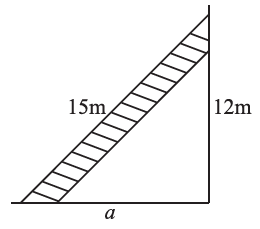12 m = leg 1 = 12 m
a = leg 2 = ?
15 m = hypotenuse = 15 m
(by Pythagoras property)
= (hyp)² = (leg 1)² + (leg 2)²
= 15² = 12² + x²
= 225 = 144 + x²
= 225 – 144
= x = 81
= √81
= 9 m
Therefore, by Pythagoras property, the distance of the foot of the ladder from the wall is 9 m.

Question 4: Which of the following can be the sides of a right triangle?
(i) 2.5 cm, 6.5 cm, 6 cm
(ii) 2 cm, 2 cm, 5 cm
(iii) 1.5 cm, 2cm, 2.5 cm
In the case of right-angled triangles, identify the right angles.
(i) Let a = 2.5 cm = leg 1
b = 6 cm = leg 2
c = 6.5 cm = hypotenuse
(by Pythagoras property)
= (hyp)² = (leg 1)² + (leg 2)²
= 6.5² = 2.5² + 6²
= 42.25 = 6.25 + 36
Therefore, by the property with these measurements we can make a triangle. The right angle is opposite to hypotenuse of the triangle.

(ii) Let a = 2 cm = leg 1
b = 2 cm = leg 2
c = 5 cm = hypotenuse
(by Pythagoras property)
= (hyp)² = (leg 1)² + (leg 2)²
= 5² = 2² + 2²
= 25 = 4 + 4
Therefore, by the property with these measurements we cannot make a triangle.

(iii) Let a = 1.5 cm = leg 1
b = 2 cm = leg 2
c = 2.5 cm = hypotenuse
(by Pythagoras property)
= (hyp)² = (leg 1)² + (leg 2)²
= 2.5² = 1.5² + 2²
= 6.25 = 2.25 + 4
Therefore, by the property with these measurements we can make a triangle. The right angle is opposite to hypotenuse of the triangle.

Question 5: A tree is broken at a height of 5 m from the ground and its top touches the ground at a distance of 12 m from the base of the tree. Find the original height of the tree.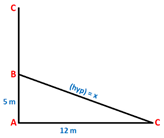AB = leg 1 = 5 m
AC = leg 2 = 12 m
BC = hypotenuse = x
(by Pythagoras property)
In ΔABC,
= (hyp)² = (leg 1)² + (leg 2)²
= x² = 5² + 12²
= x² = 25 + 144
= x² = 169
= x² = √169 = 13 m
Therefore, BC is 13 m.

Original height of the tree = AB + BC
= 5 + 13
= 18 m
Therefore, the original height of tree is 18 m.

Question 6: Angles Q and R of a ΔPQR are 25o and 65o.
Write which of the following is true:
(i) PQ2 + QR2 = RP2
(ii) PQ2 + RP2 = QR2
(iii) RP2 + QR2 = PQ2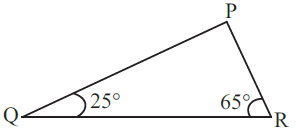(by angle sum property)
The sum of measure of angles = 180°
= 180° = x + 25° + 65°
= 180 - (25 + 65)
= 180 – 90 = 90°
Therefore, by the property angle P = 90°. The right angle is opposite to hypotenuse. So, QR is the hypotenuse.

PQ = leg 1
PR = leg 2
QR = hypotenuse
(by Pythagoras property)
= (hyp)² = (leg 1)² + (leg 2)²
= QR² = PQ² + PR²
Therefore, option 2 is correct.

Question 7: Find the perimeter of the rectangle whose length is 40 cm and a diagonal is 41 cm.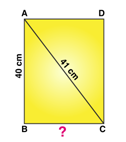AC = hypotenuse = 41 cm
BC = leg 1 = ?
AB = leg 2 = 40 cm
(by Pythagoras property)
= (hyp)² = (leg 1)² + (leg 2)²
= 41² = x² + 40²
= 1681 = x² + 1600
= 1681 – 1600 = 81
= √81 = 9 cm
Therefore, BC is 9 cm.

Length of rectangle = 40 cm
Breadth of rectangle = 9 cm
Perimeter of rectangle = 2 x (l + b)
= 2 x (40 + 9)
= 2 x 49
= 98 cm
Therefore, the perimeter of rectangle is 98 cm.

Question 8: The diagonals of a rhombus measure 16 cm and 30 cm. Find its perimeter.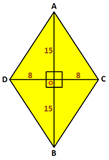Length of AC = 16 cm
Length of BD = 30 cm
△AOD is a right angled triangle.
(by Pythagoras property)
= (hyp)² = (leg 1)² + (leg 2)²
= x² = 8² + 15²
= x² = 64 + 225
= x² = 289 = √289
= x² = 17 cm

Perimeter of Rhombus = 4 x side
= 4 x 17
= 68 cm
Therefore, the perimeter of the rhombus is 68 cm.